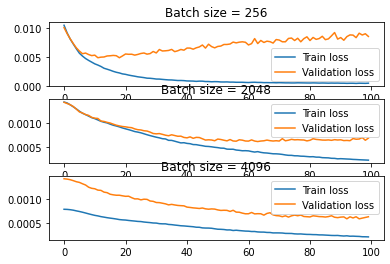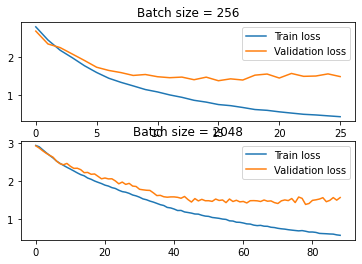Bigger batch size improves training by too much

Hello, I am writing a classifier that takes a surname and predicts a language it belongs to. I was experimenting with different batch sizes and found that small batch sizes (256 and less) perform poorly compared to big batch sizes (2048 and more). Could someone give me some insight on why this is happening? Thank you.

Training code:

def indices_to_packed(names, input_size):
names = [F.one_hot(item, input_size).float() for item in names]
names_packed = pack_sequence(names, enforce_sorted=False)
return names_packed

def infer(model, data, labels, lengths, device):
data_packed = indices_to_packed(data, model.rnn.input_size)
data_packed, labels, lengths = data_packed.to(device), labels.to(device), lengths.to(device)
preds = model(data_packed, lengths)
loss = loss_fn(preds, labels)
return loss, preds

results = {}
epochs = 100
for BATCH_SIZE in [256, 2048, 4096]:
model = LSTM(input_size, HIDDEN_SIZE, NUM_LAYERS, DROPOUT, output_size)
model.to(device)
train_losses = []
val_losses = []
cur_losses = {}
duration = 0
for epoch in range(epochs):
start = time.time()
train_loss = 0
model.train()
# Using PackedSequence
for names, langs, lengths in train_loader:
loss, _ = infer(model, names, langs, lengths, device)
loss.backward()
optimizer.step()
train_loss += loss

train_loss /= len(train_data)
train_losses.append(train_loss.cpu().detach().numpy())

model.eval()
val_loss = 0
for names, langs, lengths in val_loader:
loss, _ = infer(model, names, langs, lengths, device)
val_loss += loss
val_loss /= len(val_data)
val_losses.append(val_loss.cpu().detach().numpy())

cur_duration = time.time() - start
duration += cur_duration

log_line = (f"BATCH_SIZE: {BATCH_SIZE} epoch: {epoch} train loss: "
f"{train_loss:.5f} val loss: {val_loss:.5f}")
print(log_line)
cur_losses["train_losses"] = train_losses
cur_losses["val_losses"] = val_losses
results[BATCH_SIZE] = {"losses" : cur_losses, "duration" : duration, "model": model}

Model:

class LSTM(nn.Module):
def __init__(self, input_size, hidden_size, num_layers, dropout, output_size):
super().__init__()

self.rnn = nn.LSTM(input_size, hidden_size, num_layers, dropout=DROPOUT)
self.linear = nn.Linear(hidden_size, output_size)
self.softmax = nn.LogSoftmax(dim=1)

def forward(self, x, lengths):
lstm_out, _ = self.rnn(x)
# https://discuss.pytorch.org/t/get-each-sequences-last-item-from-packed-sequence/41118/7
sum_batch_sizes = torch.cat((
torch.zeros(2, dtype=torch.int64),
torch.cumsum(lstm_out.batch_sizes, 0)
))
sorted_lengths = lengths[lstm_out.sorted_indices]
last_seq_idxs = sum_batch_sizes[sorted_lengths] + torch.arange(lengths.size(0))
last_seq_items = lstm_out.data[last_seq_idxs]
lstm_last_out = last_seq_items[lstm_out.unsorted_indices]
linear_out = self.linear(lstm_last_out)
softmax_out = self.softmax(linear_out)

return softmax_outThe larger your batch size, the smaller on average your gradients. This also means that smaller batch size show a bit more erratic convergence.

Try lowering the learning rate for your smaller batch size.

Turns out I was calculating the losses wrong. After changing the calculation to

val_loss += loss.item() * len(names)

and